# Maximum Possible Edge Disjoint Spanning Tree From a Complete Graph

Give a complete graph with N-vertices. The task is to find out the maximum number of edge-disjoint spanning tree possible.

Edge-disjoint Spanning Tree is a spanning tree where no two trees in the set have an edge in common.

Examples:

```Input : N = 4
Output : 2

Input : N = 5
Output : 2
```

## Recommended: Please try your approach on {IDE} first, before moving on to the solution.

The maximum number of possible Edge-Disjoint Spanning tree from a complete graph with N vertices can be given as,

```Max Edge-disjoint spanning tree = floor(N / 2)
```

Let’s look at some examples:

Example 1:

Complete graph with 4 vertices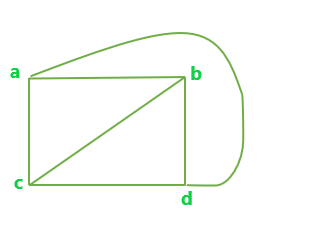All possible Edge-disjoint spanning trees for the above graph are: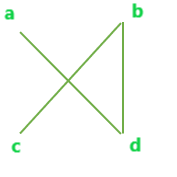A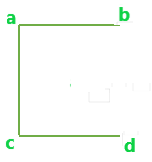B

Example 2:

Complete graph with 5 vertices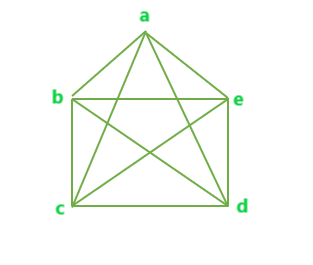All possible Edge-disjoint spanning trees for the above graph are: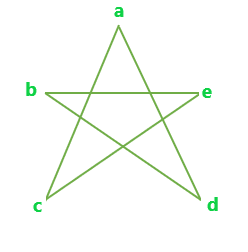A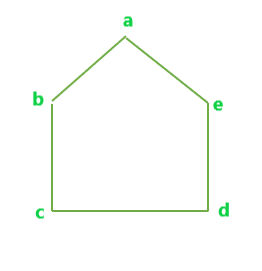B

Below is the program to find the maximum number of edge-disjoint spanning trees possible.

## C++

 `// C++ program to find the maximum number of  ` `// Edge-Disjoint Spanning tree possible ` ` `  `#include ` `using` `namespace` `std; ` ` `  `// Function to calculate max number of  ` `// Edge-Disjoint Spanning tree possible ` `float` `edgeDisjoint(``int` `n) ` `{ ` `    ``float` `result = 0; ` ` `  `    ``result = ``floor``(n / 2); ` ` `  `    ``return` `result; ` `} ` ` `  `// Driver code ` `int` `main() ` `{ ` `    ``int` `n = 4; ` ` `  `    ``cout << edgeDisjoint(n); ` ` `  `    ``return` `0; ` `} `

## Java

 `// Java program to find the maximum   ` `// number of Edge-Disjoint Spanning ` `// tree possible  ` `import` `java.io.*; ` ` `  `class` `GFG ` `{ ` `     `  `// Function to calculate max number  ` `// of Edge-Disjoint Spanning tree ` `// possible  ` `static` `double` `edgeDisjoint(``int` `n)  ` `{  ` `    ``double` `result = ``0``;  ` ` `  `    ``result = Math.floor(n / ``2``);  ` ` `  `    ``return` `result;  ` `}  ` ` `  `// Driver Code ` `public` `static` `void` `main(String[] args) ` `{ ` `    ``int` `n = ``4``;  ` `    ``System.out.println((``int``)edgeDisjoint(n)); ` `} ` `} ` ` `  `// This code is contributed ` `// by Naman_Garg `

## Python3

 `# Python 3 to find the maximum  ` `# number of Edge-Disjoint  ` `# Spanning tree possible  ` `import` `math ` ` `  `# Function to calculate max  ` `# number of Edge-Disjoint  ` `# Spanning tree possible  ` `def` `edgeDisjoint(n): ` ` `  `    ``result ``=` `0` ` `  `    ``result ``=` `math.floor(n ``/` `2``)  ` ` `  `    ``return` `result  ` ` `  `# Driver Code ` `if` `__name__ ``=``=` `"__main__"` `:  ` ` `  `    ``n ``=` `4` ` `  `    ``print``(``int``(edgeDisjoint(n))) ` ` `  `# This Code is contributed ` `# by Naman_Garg `

## C#

 `// C# program to find the maximum number of  ` `// Edge-Disjoint Spanning tree possible  ` `using` `System;  ` ` `  `class` `GFG  ` `{  ` ` `  `// Function to calculate max number of  ` `// Edge-Disjoint Spanning tree possible  ` `static` `double` `edgeDisjoint(``double` `n)  ` `{  ` `    ``double` `result = 0;  ` ` `  `    ``result = Math.Floor(n / 2);  ` ` `  `    ``return` `result;  ` `}  ` ` `  `// Driver Code  ` `public` `static` `void` `Main()  ` `{  ` `    ``int` `n = 4; ` ` `  `    ``Console.Write(edgeDisjoint(n));  ` `}  ` `}  ` ` `  `// This code is contributed  ` `// by Sanjit_Prasad  `

## PHP

 ` `

Output:

```2
```

Don’t stop now and take your learning to the next level. Learn all the important concepts of Data Structures and Algorithms with the help of the most trusted course: DSA Self Paced. Become industry ready at a student-friendly price.

My Personal Notes arrow_drop_upCheck out this Author's contributed articles.

If you like GeeksforGeeks and would like to contribute, you can also write an article using contribute.geeksforgeeks.org or mail your article to contribute@geeksforgeeks.org. See your article appearing on the GeeksforGeeks main page and help other Geeks.

Please Improve this article if you find anything incorrect by clicking on the "Improve Article" button below.

Article Tags :
Practice Tags :

Be the First to upvote.

Please write to us at contribute@geeksforgeeks.org to report any issue with the above content.